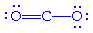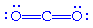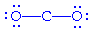# Octet Rule

Is this how you tell when to form double bonds when drawing lewis structures, such as with CO2?

And a somewhat related question, how do we know that C,N,O,F all have four orbitals?

Have you studied anything about formal charges? You have to know how formal charges work in order to draw CO2. There are a few ways you can draw CO2 to obey the octet rule but which one is the correct one?

I'm not sure what you mean by four orbitals. Is this a question from your book?

Last edited:
I know formal charges. How does that help?

Do you know what the formal charges of Oxygen and Carbon are? Add up the charges and see if it equals the total formal charge of CO2.

The problem could have been written something along the lines of CO2 or CO2^-2. The formula you just wrote did not have a formal charge so your Lewis structure should have a total formal charge of zero.

Yeah but if it says with an FC of 0, don't you just base your structure off of fulfilling the octet rule?

Okay, let me rephrase that. You have to know your formal charges in case you get one of these.2nd one since the others don't fulfill the octet rule?

2nd one since the others don't fulfill the octet rule?

Yeah but not solely on the octet rule. If you were asked to draw CO2^-2 or CO2^-1, could you draw them? Formal charges is the best way to get the correct result.

Right, but you use the octet rule if the FC is 0, correct?

Also, don't they need to tell you which atom has the formal charge :O If it says CO2 with a formal charge of -2, what do you do? do they mean the O has a charge of -2?

They don't need to tell you anything because it's already written in the equation. The whole compound CO2 has a formal charge of zero. If they don't write the charge on the equation then you can assumes a total charge of zero. It's something that you must know right off the bat. First you do the formal charge of both Oxygen and the Carbon. Then, add up the charges and you should get zero.

Thanks, but still wondering about an answer to my question. If they say it has a -2 charge, do you do that for the C or for the O? C right?

And for FC 0, you just do the octet rule correct?

Thanks, but still wondering about an answer to my question. If they say it has a -2 charge, do you do that for the C or for the O? C right?

And for FC 0, you just do the octet rule correct?

I guess it's a little hard to explain since I can't show you. They will never say the total formal charge of CO2 is -2. They will just write the equation CO2^-2. The problem you posted earlier was CO2, so you assume that the total charge is zero. The charge at the end of the equation CO2^-2 belongs to the whole compound.

For your second question, yeah I think that could work. I don't know any cases off hand that it wouldn't for Ochem. In Gen Chem, it doesn't work that way. In Ochem, you will be given reactions with charges. So it is important that you know where they come from in order for you to draw them correctly.

Last edited:
So how would you go about drawing CO2^2- then?

It's one of those pictures I posted up there. See if you can figure it out. The only way to go about it is using formal charges.

The -2 charge is on the C or the O....?

You know what man? I just checked over all those pictures and all of them have a formal charge of zero. You can just go buy the octet rule on that. My fault.:shy:

Last edited:
And a somewhat related question, how do we know that C,N,O,F all have four orbitals?

Looked them up they all have 3.

If you are referring to the s,p,d,f orbitals that theoretically any atom can have if charged enough, than yes they have 4.

Why is it that for example SO4 2- 'breaks' the octet rule and engages a 'hypervalent bonding'?

I'm not sure if there is something to really understand, it's just a rule that applies to certain elements. The rule is applicable to the main-group elements, especially carbon, nitrogen, oxygen, and the halogens, but also to metals such as sodium or magnesium.

http://en.wikipedia.org/wiki/Octet_rule